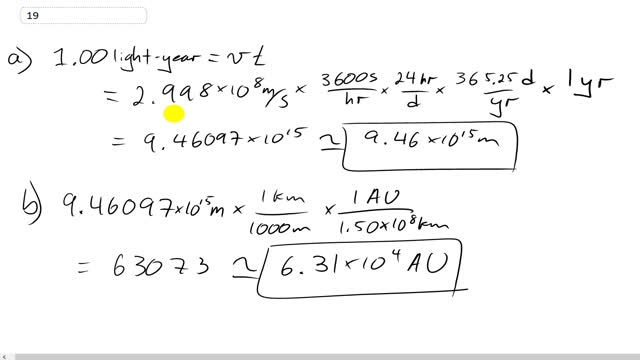## You are here

Question:

A light-year is the distance light travels in one year (at speed $= 2.998 \times 10^8 \textrm{ m/s}$).

1. How many meters are there in 1.00 light-year?
2. An astronomical unit (AU) is the average distance from the Sun to Earth, $1.50 \times 10^8 \textrm{ km}$. How many AU are there in 1.00 light-year?

Giancoli, Douglas C., Physics: Principles with Applications, 7th Ed., ©2014. Reprinted by permission of Pearson Education Inc., New York.
The question will be visible after logging in, as required by Pearson Education Inc.

a) $9.46 \times 10^{15} \textrm{ m}$
b) $6.31 \times 10^4 \textrm{ AU}$